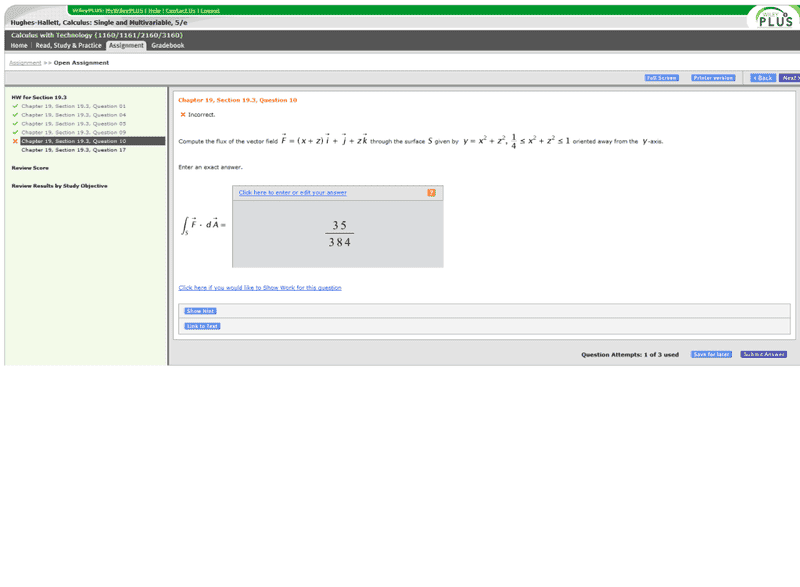# Flux Integral

## Homework Statement## Homework Equations

I know that flux is ∫∫F[r(t,s)]dotted[∂r/∂s x ∂r/∂t]dtds

## The Attempt at a Solution

So I parameterized the equation y=x^2+z^2

as:

x = s
y = s^2 + t^2
z = t

r(t,s) = [s, s^2+t^2, t]
I take the partial derivative of r with respect to s and t and then take the cross product.

I then that F[r(s,t)] and dot that with the cross product above.

I then need the boundaries which I find to be 1/sqrt(2) ≤ s ≤ 1/sqrt(8) and 1/sqrt(2) ≤ s ≤ 1/sqrt(8)

After integrating I get the answer 35/384 which says it's incorrect. I did the problem all over again and got the same exact answer. Any help?

## Answers and Replies

LCKurtz
Science Advisor
Homework Helper
Gold Member
Have you checked whether ##r_s\times r_t## is in the direction consistent with the given orientation of the surface? Also, just curious, why you rename the variables instead of just using ##x## and ##z## as the parameters.

HallsofIvy
Science Advisor
Homework Helper
Doing this problem in the same way you describe, I get $(21/16)\pi$ rather than your answer. You will need to describe more exactly what you did. What did you get for $d\vec{S}$? What limits of integration did you use?

LCKurtz, my rs×rt does point in the direction I believe it is supposed to. Also, the reason I renamed the variables was just my preference I guess. When setting up the vector, I like to think of each component that acts like the x, y, and z. It's probably unnecessary but that what I did.

Let me give more detail into my work.

- So I parameterized the equation y=x^2+z^2

x = s
y = s^2 + t^2
z = t

- I then get r(t,s) = [s, s^2 + t^2, t]

- I then take the partial derivative with respect to both variables
∂r/∂s = [1, 2s, 0] and ∂r/∂t = [0, 2t, 1]

- I then take the cross product of these to get,

[2s, -1, 2t]

- Now this cross product is oriented away from the y axis at all times, unless t and s are 0 I suppose...

- I then use this formula ∫∫F[r(t,s)]dotted[∂r/∂s x ∂r/∂t]dtds

- ∫∫ [s+t, 1, t]dotted[2s,-1,2t]dtds

-Which equals

∫∫(2t^2+2ts+2s^2-1)dtds

-I use the limits of 1/sqrt(2) ≤ s ≤ 1/sqrt(8) and 1/sqrt(2) ≤ s ≤ 1/sqrt(8) because these translate to match up with my parametrized equations and the limitation that 1/4 ≤ y≤ 1

LCKurtz
Science Advisor
Homework Helper
Gold Member
Ignore that post I just did if you saw it. Be back later.

LCKurtz
Science Advisor
Homework Helper
Gold Member
∫∫(2t^2+2ts+2s^2-1)dtds

-I use the limits of 1/sqrt(2) ≤ s ≤ 1/sqrt(8) and 1/sqrt(2) ≤ s ≤ 1/sqrt(8) because these translate to match up with my parametrized equations and the limitation that 1/4 ≤ y≤ 1

You are OK to there. But those limits describe a square. You need to change the integral to polar coordinates. Your t and s parameters should describe the region between two circles in the st (same as xz) plane.

In doing that,

would the ∫∫(2t^2+2ts+2s^2-1)dtds turn into ∫∫((2r^2+2*r*sin(θ)*r*cos(θ)-1)*r)drdθ with boundaries of 1/4≤ r ≤ 1 and 0 ≤ θ ≤ 2pi ?

LCKurtz
Science Advisor
Homework Helper
Gold Member
In doing that,

would the ∫∫(2t^2+2ts+2s^2-1)dtds turn into ∫∫((2r^2+2*r*sin(θ)*r*cos(θ)-1)*r)drdθ with boundaries of 1/4≤ r ≤ 1 and 0 ≤ θ ≤ 2pi ?

Those are the limits for ##r^2##, not ##r##.

Those are the limits for ##r^2##, not ##r##.

Ahh, yes I almost messed that on up big time. So it should be 1/2 ≤ r ≤ 1

Thanks so much LCKurtz, that seemed to be what I was doing wrong and I got the correct answer.